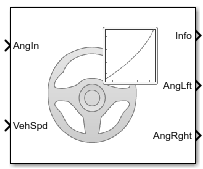# Mapped Steering

Mapped steering with speed-dependent option

• Library:
• Vehicle Dynamics Blockset / Steering

•## Description

The Mapped Steering block implements lookup tables to calculate the right and left wheel angles. Use the Speed dependent parameter to implement a speed-dependent table for the angle calculations. The block uses the vehicle coordinate system.

Speed DependentImplementationCalculations
`on` (default)

Block uses three tables:

• fs — Function of vehicle speed

• fL — Function of superimposed steering wheel angle

• fR — Function of superimposed steering wheel angle

`$\begin{array}{l}{\delta }_{SpdF}={f}_{s}\left(v\right)\\ {\delta }_{SuprImp}={\delta }_{SpdF}\cdot {\delta }_{in}\\ \\ {\delta }_{L}={f}_{L}\left({\delta }_{SuprImp}\right)\\ {\delta }_{R}={f}_{R}\left({\delta }_{SuprImp}\right)\end{array}$`

`off`

Block uses two tables:

• fL — Function of steering wheel angle

• fR — Function of steering wheel angle

`$\begin{array}{l}{\delta }_{L}={f}_{L}\left({\delta }_{in}\right)\\ {\delta }_{R}={f}_{R}\left({\delta }_{in}\right)\end{array}$`

The equations use these variables.

 δin Steering wheel angle δSpdF Steering wheel angle speed factor δSuprImp Superimposed steering wheel angle δL, δR Left and right wheel angles, respectively

## Ports

### Input

expand all

Vehicle speed, Vehspd, in m/s.

#### Dependencies

To enable this port, select Speed dependent.

### Output

expand all

Bus signal containing these block calculations.

SignalDescriptionVariableUnit

`AngLft`

Left wheel angle

δL

`AngRght`

Left wheel angle

δR

Left wheel angle, δL, in rad.

Right wheel angle, δR, in rad.

## Parameters

expand all

Left wheel angle table, δL, in rad.

Right wheel angle table, δR, in rad.

Vehicle speed breakpoints, in m/s.

#### Dependencies

To create this parameter, select Speed dependent.

Superimposed speed factor table, ƒs, dimensionless. The table is a factor of vehicle speed, v.

#### Dependencies

To create this parameter, select Speed dependent.

 Crolla, David, David Foster, et al. Encyclopedia of Automotive Engineering. Volume 4, Part 5 (Chassis Systems) and Part 6 (Electrical and Electronic Systems). Chichester, West Sussex, United Kingdom: John Wiley & Sons Ltd, 2015.

 Gillespie, Thomas. Fundamentals of Vehicle Dynamics. Warrendale, PA: Society of Automotive Engineers, 1992.

 Vehicle Dynamics Standards Committee. Vehicle Dynamics Terminology. SAE J670. Warrendale, PA: Society of Automotive Engineers, 2008.

## SupportGet trial now